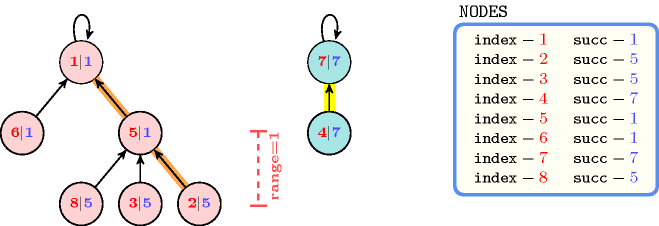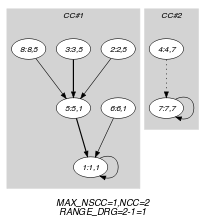## 5.404. tree_range

Origin

Derived from $\mathrm{𝚝𝚛𝚎𝚎}$.

Constraint

$\mathrm{𝚝𝚛𝚎𝚎}_\mathrm{𝚛𝚊𝚗𝚐𝚎}\left(\mathrm{𝙽𝚃𝚁𝙴𝙴𝚂},𝚁,\mathrm{𝙽𝙾𝙳𝙴𝚂}\right)$

Arguments
 $\mathrm{𝙽𝚃𝚁𝙴𝙴𝚂}$ $\mathrm{𝚍𝚟𝚊𝚛}$ $𝚁$ $\mathrm{𝚍𝚟𝚊𝚛}$ $\mathrm{𝙽𝙾𝙳𝙴𝚂}$ $\mathrm{𝚌𝚘𝚕𝚕𝚎𝚌𝚝𝚒𝚘𝚗}\left(\mathrm{𝚒𝚗𝚍𝚎𝚡}-\mathrm{𝚒𝚗𝚝},\mathrm{𝚜𝚞𝚌𝚌}-\mathrm{𝚍𝚟𝚊𝚛}\right)$
Restrictions
 $\mathrm{𝙽𝚃𝚁𝙴𝙴𝚂}\ge 0$ $𝚁\ge 0$ $𝚁<|\mathrm{𝙽𝙾𝙳𝙴𝚂}|$ $|\mathrm{𝙽𝙾𝙳𝙴𝚂}|>0$ $\mathrm{𝚛𝚎𝚚𝚞𝚒𝚛𝚎𝚍}$$\left(\mathrm{𝙽𝙾𝙳𝙴𝚂},\left[\mathrm{𝚒𝚗𝚍𝚎𝚡},\mathrm{𝚜𝚞𝚌𝚌}\right]\right)$ $\mathrm{𝙽𝙾𝙳𝙴𝚂}.\mathrm{𝚒𝚗𝚍𝚎𝚡}\ge 1$ $\mathrm{𝙽𝙾𝙳𝙴𝚂}.\mathrm{𝚒𝚗𝚍𝚎𝚡}\le |\mathrm{𝙽𝙾𝙳𝙴𝚂}|$ $\mathrm{𝚍𝚒𝚜𝚝𝚒𝚗𝚌𝚝}$$\left(\mathrm{𝙽𝙾𝙳𝙴𝚂},\mathrm{𝚒𝚗𝚍𝚎𝚡}\right)$ $\mathrm{𝙽𝙾𝙳𝙴𝚂}.\mathrm{𝚜𝚞𝚌𝚌}\ge 1$ $\mathrm{𝙽𝙾𝙳𝙴𝚂}.\mathrm{𝚜𝚞𝚌𝚌}\le |\mathrm{𝙽𝙾𝙳𝙴𝚂}|$
Purpose

Cover the digraph $G$ described by the $\mathrm{𝙽𝙾𝙳𝙴𝚂}$ collection with $\mathrm{𝙽𝚃𝚁𝙴𝙴𝚂}$ trees in such a way that each vertex of $G$ belongs to one distinct tree. $𝚁$ is the difference between the longest and the shortest paths (from a leaf to a root) of the final graph.

Example
$\left(\begin{array}{c}2,1,〈\begin{array}{cc}\mathrm{𝚒𝚗𝚍𝚎𝚡}-1\hfill & \mathrm{𝚜𝚞𝚌𝚌}-1,\hfill \\ \mathrm{𝚒𝚗𝚍𝚎𝚡}-2\hfill & \mathrm{𝚜𝚞𝚌𝚌}-5,\hfill \\ \mathrm{𝚒𝚗𝚍𝚎𝚡}-3\hfill & \mathrm{𝚜𝚞𝚌𝚌}-5,\hfill \\ \mathrm{𝚒𝚗𝚍𝚎𝚡}-4\hfill & \mathrm{𝚜𝚞𝚌𝚌}-7,\hfill \\ \mathrm{𝚒𝚗𝚍𝚎𝚡}-5\hfill & \mathrm{𝚜𝚞𝚌𝚌}-1,\hfill \\ \mathrm{𝚒𝚗𝚍𝚎𝚡}-6\hfill & \mathrm{𝚜𝚞𝚌𝚌}-1,\hfill \\ \mathrm{𝚒𝚗𝚍𝚎𝚡}-7\hfill & \mathrm{𝚜𝚞𝚌𝚌}-7,\hfill \\ \mathrm{𝚒𝚗𝚍𝚎𝚡}-8\hfill & \mathrm{𝚜𝚞𝚌𝚌}-5\hfill \end{array}〉\hfill \end{array}\right)$

The $\mathrm{𝚝𝚛𝚎𝚎}_\mathrm{𝚛𝚊𝚗𝚐𝚎}$ constraint holds since the graph associated with the items of the $\mathrm{𝙽𝙾𝙳𝙴𝚂}$ collection corresponds to two trees (i.e., $\mathrm{𝙽𝚃𝚁𝙴𝙴𝚂}=2$): each tree respectively involves the vertices $\left\{1,2,3,5,6,8\right\}$ and $\left\{4,7\right\}$. Furthermore $𝚁=1$ is set to the difference between the longest path (for instance $2\to 5\to 1$) and the shortest path (for instance $4\to 7$) from a leaf to a root. Figure 5.404.1 provides the two trees associated with the example.

##### Figure 5.404.1. The two trees corresponding to the Example slot; each vertex contains the information $\mathrm{𝚒𝚗𝚍𝚎𝚡}|\mathrm{𝚜𝚞𝚌𝚌}$ where $\mathrm{𝚜𝚞𝚌𝚌}$ is the index of its father in the tree (by convention the father of the root is the root itself); the longest and shortest paths from a leaf to a root are respectively shown by thick orange and yellow line segments and have a length of 2 and 1; consequently the range is equal to 1.Typical
 $\mathrm{𝙽𝚃𝚁𝙴𝙴𝚂}<|\mathrm{𝙽𝙾𝙳𝙴𝚂}|$ $|\mathrm{𝙽𝙾𝙳𝙴𝚂}|>2$
Symmetry

Items of $\mathrm{𝙽𝙾𝙳𝙴𝚂}$ are permutable.

Arg. properties
• Functional dependency: $\mathrm{𝙽𝚃𝚁𝙴𝙴𝚂}$ determined by $\mathrm{𝙽𝙾𝙳𝙴𝚂}$.

• Functional dependency: $𝚁$ determined by $\mathrm{𝙽𝙾𝙳𝙴𝚂}$.

Reformulation

By introducing a distance variable ${D}_{i}$, an occurrence variable ${O}_{i}$ and a leave variable ${L}_{i}$ $\left(1\le i\le |\mathrm{𝙽𝙾𝙳𝙴𝚂}|\right)$ for each item $i$ of the $\mathrm{𝙽𝙾𝙳𝙴𝚂}$ collection, where:

• ${D}_{i}$ represents the number of vertices from $i$ to the root of the corresponding tree,

• ${O}_{i}$ gives the number of occurrences of value $i$ within variables $\mathrm{𝙽𝙾𝙳𝙴𝚂}\left[1\right].\mathrm{𝚜𝚞𝚌𝚌},\mathrm{𝙽𝙾𝙳𝙴𝚂}\left[2\right].\mathrm{𝚜𝚞𝚌𝚌},\cdots ,\mathrm{𝙽𝙾𝙳𝙴𝚂}\left[n\right].\mathrm{𝚜𝚞𝚌𝚌}$,

• ${L}_{i}$ is set to 1 if item $i$ corresponds to a leave (i.e., ${O}_{i}>0$) and 0 otherwise,

the $\mathrm{𝚝𝚛𝚎𝚎}_\mathrm{𝚛𝚊𝚗𝚐𝚎}$$\left(\mathrm{𝙽𝚃𝚁𝙴𝙴𝚂},𝚁,\mathrm{𝙽𝙾𝙳𝙴𝚂}\right)$ constraint can be expressed in term of a conjunction of one $\mathrm{𝚝𝚛𝚎𝚎}$ constraint, $|\mathrm{𝙽𝙾𝙳𝙴𝚂}|$ $\mathrm{𝚎𝚕𝚎𝚖𝚎𝚗𝚝}$ constraints, $|\mathrm{𝙽𝙾𝙳𝙴𝚂}|$ linear constraints, one $\mathrm{𝚐𝚕𝚘𝚋𝚊𝚕}_\mathrm{𝚌𝚊𝚛𝚍𝚒𝚗𝚊𝚕𝚒𝚝𝚢}$ constraint, $|\mathrm{𝙽𝙾𝙳𝙴𝚂}|$ reified constraints, one $\mathrm{𝚘𝚙𝚎𝚗}_\mathrm{𝚖𝚒𝚗𝚒𝚖𝚞𝚖}$, one $\mathrm{𝚖𝚊𝚡𝚒𝚖𝚞𝚖}$ and one linear constraint, where:

• The $\mathrm{𝚝𝚛𝚎𝚎}$ constraint models the fact that we have a forest of $\mathrm{𝙽𝚃𝚁𝙴𝙴𝚂}$ trees.

• Each $\mathrm{𝚎𝚕𝚎𝚖𝚎𝚗𝚝}$ constraint provides the link between the attribute $\mathrm{𝚜𝚞𝚌𝚌}$ of the $i$-th item and the distance variable ${D}_{\mathrm{𝙽𝙾𝙳𝙴𝚂}\left[i\right].\mathrm{𝚜𝚞𝚌𝚌}}$ associated with item $\mathrm{𝙽𝙾𝙳𝙴𝚂}\left[i\right].\mathrm{𝚜𝚞𝚌𝚌}$.

• Each linear constraint associated with the $i$-th item states that the difference between the distance variable ${D}_{i}$ and the distance variable ${D}_{\mathrm{𝙽𝙾𝙳𝙴𝚂}\left[i\right].\mathrm{𝚜𝚞𝚌𝚌}}$ is equal to 1.

• The $\mathrm{𝚐𝚕𝚘𝚋𝚊𝚕}_\mathrm{𝚌𝚊𝚛𝚍𝚒𝚗𝚊𝚕𝚒𝚝𝚢}$ constraint provides the number of occurrences ${O}_{i}$ of value $i$ $\left(1\le i\le |\mathrm{𝙽𝙾𝙳𝙴𝚂}|\right)$ within variables $\mathrm{𝙽𝙾𝙳𝙴𝚂}\left[1\right].\mathrm{𝚜𝚞𝚌𝚌},\mathrm{𝙽𝙾𝙳𝙴𝚂}\left[2\right].\mathrm{𝚜𝚞𝚌𝚌},\cdots ,\mathrm{𝙽𝙾𝙳𝙴𝚂}\left[|\mathrm{𝙽𝙾𝙳𝙴𝚂}|\right].\mathrm{𝚜𝚞𝚌𝚌}$. Note that, when ${O}_{i}$ is equal to 0, the corresponding $i$-th item is a leave of one of the $\mathrm{𝙽𝚃𝚁𝙴𝙴𝚂}$ trees.

• Each reified constraint of the form ${L}_{i}⇔{O}_{i}>0$ makes the link between the $i$-th occurrence variable ${O}_{i}$ and the $i$-th leave variable ${L}_{i}$.

• The $\mathrm{𝚘𝚙𝚎𝚗}_\mathrm{𝚖𝚒𝚗𝚒𝚖𝚞𝚖}$ constraint computes the minimum distance $\mathrm{𝙼𝙸𝙽}$ from the leaves to the corresponding roots. The leave variable ${L}_{i}$ is used in order to select only the distance variables corresponding to leaves.

• The $\mathrm{𝚖𝚊𝚡𝚒𝚖𝚞𝚖}$ constraint computes the maximum distance $\mathrm{𝙼𝙰𝚇}$ from the vertices to the roots. Since the maximum is achieved by a leave we do not need to focus just on the leaves as it was the case for the minimum distance $\mathrm{𝙼𝙸𝙽}$.

• The linear constraint $\mathrm{𝙼𝙰𝚇}-\mathrm{𝙼𝙸𝙽}=𝚁$ links together argument $𝚁$ to the minimum and maximum distances.

With respect to the Example slot we get the following conjunction of constraints:

$\mathrm{𝚝𝚛𝚎𝚎}$$\left(2,〈\mathrm{𝚒𝚗𝚍𝚎𝚡}-1\mathrm{𝚜𝚞𝚌𝚌}-1,\mathrm{𝚒𝚗𝚍𝚎𝚡}-2\mathrm{𝚜𝚞𝚌𝚌}-5,$

$\mathrm{𝚒𝚗𝚍𝚎𝚡}-3\mathrm{𝚜𝚞𝚌𝚌}-5,\mathrm{𝚒𝚗𝚍𝚎𝚡}-4\mathrm{𝚜𝚞𝚌𝚌}-7,$

$\mathrm{𝚒𝚗𝚍𝚎𝚡}-5\mathrm{𝚜𝚞𝚌𝚌}-1,\mathrm{𝚒𝚗𝚍𝚎𝚡}-6\mathrm{𝚜𝚞𝚌𝚌}-1,$

$\mathrm{𝚒𝚗𝚍𝚎𝚡}-7\mathrm{𝚜𝚞𝚌𝚌}-7,\mathrm{𝚒𝚗𝚍𝚎𝚡}-8\mathrm{𝚜𝚞𝚌𝚌}-5〉\right)$,

$\mathrm{𝚍𝚘𝚖𝚊𝚒𝚗}$$\left(〈{D}_{1},{D}_{2},{D}_{3},{D}_{4},{D}_{5},{D}_{6},{D}_{7},{D}_{8}〉,0,8\right)$,

$D{S}_{1}\in \left[0,8\right]$,  $\mathrm{𝚎𝚕𝚎𝚖𝚎𝚗𝚝}$$\left(1,〈0,{D}_{2},{D}_{3},{D}_{4},{D}_{5},{D}_{6},{D}_{7},{D}_{8}〉,D{S}_{1}\right)$,  ${D}_{1}-0=1$,

$D{S}_{2}\in \left[0,8\right]$,  $\mathrm{𝚎𝚕𝚎𝚖𝚎𝚗𝚝}$$\left(5,〈1,0,{D}_{3},{D}_{4},{D}_{5},{D}_{6},{D}_{7},{D}_{8}〉,D{S}_{2}\right)$,  ${D}_{2}-{D}_{5}=1$,

$D{S}_{3}\in \left[0,8\right]$,  $\mathrm{𝚎𝚕𝚎𝚖𝚎𝚗𝚝}$$\left(5,〈1,{D}_{2},0,{D}_{4},{D}_{5},{D}_{6},{D}_{7},{D}_{8}〉,D{S}_{3}\right)$,  ${D}_{3}-{D}_{5}=1$,

$D{S}_{4}\in \left[0,8\right]$,  $\mathrm{𝚎𝚕𝚎𝚖𝚎𝚗𝚝}$$\left(7,〈1,{D}_{2},{D}_{3},0,{D}_{5},{D}_{6},{D}_{7},{D}_{8}〉,D{S}_{4}\right)$,  ${D}_{4}-{D}_{7}=1$,

$D{S}_{5}\in \left[0,8\right]$,  $\mathrm{𝚎𝚕𝚎𝚖𝚎𝚗𝚝}$$\left(1,〈1,{D}_{2},{D}_{3},{D}_{4},0,{D}_{6},{D}_{7},{D}_{8}〉,D{S}_{5}\right)$,  ${D}_{5}-1=1$,

$D{S}_{6}\in \left[0,8\right]$,  $\mathrm{𝚎𝚕𝚎𝚖𝚎𝚗𝚝}$$\left(1,〈1,3,3,{D}_{4},2,0,{D}_{7},{D}_{8}〉,D{S}_{6}\right)$,  ${D}_{6}-1=1$,

$D{S}_{7}\in \left[0,8\right]$,  $\mathrm{𝚎𝚕𝚎𝚖𝚎𝚗𝚝}$$\left(7,〈1,3,3,{D}_{4},2,2,0,{D}_{8}〉,D{S}_{7}\right)$,  ${D}_{7}-0=1$,

$D{S}_{8}\in \left[0,8\right]$,  $\mathrm{𝚎𝚕𝚎𝚖𝚎𝚗𝚝}$$\left(5,〈1,3,3,2,2,2,1,0〉,D{S}_{8}\right)$,  ${D}_{8}-2=1$,

$\mathrm{𝚐𝚕𝚘𝚋𝚊𝚕}_\mathrm{𝚌𝚊𝚛𝚍𝚒𝚗𝚊𝚕𝚒𝚝𝚢}$$\left(〈1,5,5,7,1,1,7,5〉,〈\mathrm{𝚟𝚊𝚕}-1\mathrm{𝚗𝚘𝚌𝚌𝚞𝚛𝚛𝚎𝚗𝚌𝚎}-3,$

$\mathrm{𝚟𝚊𝚕}-2\mathrm{𝚗𝚘𝚌𝚌𝚞𝚛𝚛𝚎𝚗𝚌𝚎}-0,$

$\mathrm{𝚟𝚊𝚕}-3\mathrm{𝚗𝚘𝚌𝚌𝚞𝚛𝚛𝚎𝚗𝚌𝚎}-0,$

$\mathrm{𝚟𝚊𝚕}-4\mathrm{𝚗𝚘𝚌𝚌𝚞𝚛𝚛𝚎𝚗𝚌𝚎}-0,$

$\mathrm{𝚟𝚊𝚕}-5\mathrm{𝚗𝚘𝚌𝚌𝚞𝚛𝚛𝚎𝚗𝚌𝚎}-3,$

$\mathrm{𝚟𝚊𝚕}-6\mathrm{𝚗𝚘𝚌𝚌𝚞𝚛𝚛𝚎𝚗𝚌𝚎}-0,$

$\mathrm{𝚟𝚊𝚕}-7\mathrm{𝚗𝚘𝚌𝚌𝚞𝚛𝚛𝚎𝚗𝚌𝚎}-2,$

$\mathrm{𝚟𝚊𝚕}-8\mathrm{𝚗𝚘𝚌𝚌𝚞𝚛𝚛𝚎𝚗𝚌𝚎}-0〉\right)$,

$1⇔3>0,0⇔0>0,0⇔0>0,0⇔0>0,$

$1⇔3>0,0⇔0>0,1⇔2>0,0⇔0>0$,

$\mathrm{𝚘𝚙𝚎𝚗}_\mathrm{𝚖𝚒𝚗𝚒𝚖𝚞𝚖}$$\left(\mathrm{𝙼𝙸𝙽},〈\mathrm{𝚟𝚊𝚛}-3\mathrm{𝚋𝚘𝚘𝚕}-1,\mathrm{𝚟𝚊𝚛}-0\mathrm{𝚋𝚘𝚘𝚕}-0,$

$\mathrm{𝚟𝚊𝚛}-0\mathrm{𝚋𝚘𝚘𝚕}-0,\mathrm{𝚟𝚊𝚛}-0\mathrm{𝚋𝚘𝚘𝚕}-0,$

$\mathrm{𝚟𝚊𝚛}-3\mathrm{𝚋𝚘𝚘𝚕}-1,\mathrm{𝚟𝚊𝚛}-0\mathrm{𝚋𝚘𝚘𝚕}-0,$

$\mathrm{𝚟𝚊𝚛}-2\mathrm{𝚋𝚘𝚘𝚕}-1,\mathrm{𝚟𝚊𝚛}-0\mathrm{𝚋𝚘𝚘𝚕}-0〉\right)$,

$\mathrm{𝚖𝚊𝚡𝚒𝚖𝚞𝚖}$$\left(\mathrm{𝙼𝙰𝚇},〈1,3,3,2,2,2,1,3〉\right)$,

$\mathrm{𝙼𝙰𝚇}-\mathrm{𝙼𝙸𝙽}=𝚁=1$.

related: $\mathrm{𝚋𝚊𝚕𝚊𝚗𝚌𝚎}$ (balanced tree versus balanced assignment).

Keywords
Arc input(s)

$\mathrm{𝙽𝙾𝙳𝙴𝚂}$

Arc generator
$\mathrm{𝐶𝐿𝐼𝑄𝑈𝐸}$$↦\mathrm{𝚌𝚘𝚕𝚕𝚎𝚌𝚝𝚒𝚘𝚗}\left(\mathrm{𝚗𝚘𝚍𝚎𝚜}\mathtt{1},\mathrm{𝚗𝚘𝚍𝚎𝚜}\mathtt{2}\right)$

Arc arity
Arc constraint(s)
$\mathrm{𝚗𝚘𝚍𝚎𝚜}\mathtt{1}.\mathrm{𝚜𝚞𝚌𝚌}=\mathrm{𝚗𝚘𝚍𝚎𝚜}\mathtt{2}.\mathrm{𝚒𝚗𝚍𝚎𝚡}$
Graph property(ies)
 $•$$\mathrm{𝐌𝐀𝐗}_\mathrm{𝐍𝐒𝐂𝐂}$$\le 1$ $•$$\mathrm{𝐍𝐂𝐂}$$=\mathrm{𝙽𝚃𝚁𝙴𝙴𝚂}$ $•$$\mathrm{𝐑𝐀𝐍𝐆𝐄}_\mathrm{𝐃𝐑𝐆}$$=𝚁$

Graph model

Parts (A) and (B) of Figure 5.404.2 respectively show the initial and final graph associated with the Example slot. Since we use the $\mathrm{𝚁𝙰𝙽𝙶𝙴}_\mathrm{𝙳𝚁𝙶}$ graph property, we respectively display the longest and shortest paths of the final graph with a bold and a dash line.

##### Figure 5.404.2. Initial and final graph of the $\mathrm{𝚝𝚛𝚎𝚎}_\mathrm{𝚛𝚊𝚗𝚐𝚎}$ constraint(a) (b)